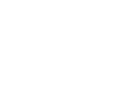Share

# How to Draw or Plot a Demand Curve on a GraphWhen a president or prime minister talks about easing of monetary policy or fiscal expansion to stimulate the economy, they are talking about changes to the aggregate demand curve. Aggregate demand is the sum of individual demand curves of all buyers inside and outside of a country.An individual demand curve represents the quantity of a commodity that a consumer is willing to buy based on price in graph form. For normal, daily goods, there is an inverse or negative relationship between the desired quantity and the price. In other words, consumers buy more commodities at lower prices than at higher prices. This microeconomic relationship is known as the law of demand.

In this oneHOWTO article we'll show you how to draw or plot a demand curve on a graph so that you can understand the concept better, and perhaps apply it to your own business.

You'll need:
• Ruler (optional)
• Graph paper (optional)
You may also be interested in: How to Calculate MPC in Macroeconomics
Steps to follow:
1

The first step to draw or plot a demand curve on a graph is to start with the basic grid. This means you have to create a table with two columns, one for price and one for quantity.

This kind of demand curve on a graph works for a single, daily commodity. In this example, we'll be talking about cheeseburgers.

2

Once you have the grid for the demand curve on a graph, fill in the columns or axes with the amount of product that is available to be bought at different prices.

Enter prices ranging from 1 to 10 dollars - or your own currency, whatever it is - with an increase of 1 dollar in the price column. Enter the corresponding number of cheeseburgers you want to buy at each price in the quantity column.

3

Draw a vertical line - the axis - with a ruler. Label it with prices or P (y). Enter each price on the axis line with the lowest price at the lowest end of the line. Increase the prices on the line until it reaches its highest price.

4

Draw a horizontal line - the x axis - from the foot of the vertical line (y axis) and towards the right. Label it with quantity or Q (x).

Write each commodity amount below the x axis line, with the lowest amount at the corner where the x axis and y axis meet. Increase the amounts along the line, moving to the right, until you reach your highest amount.

5

Enter the desired quantity at the first price with a dot on the graph. Start from the top of the demand curve.

If you want 10 cheeseburgers at 1 dollar each, the mark on the x axis is equal to 10 and on the Y axis is equal to 1. That is at (10.1) in the graph.

6

Repeat step 5 for each price and amount.

7

Join the dots to complete the demand curve.

8

This is how to draw or plot a demand curve on a graph. If you have any tips or questions, tell us in the comments section!

If you want to read similar articles to How to Draw or Plot a Demand Curve on a Graph, we recommend you visit our Economy & business category.

## Write a comment